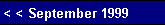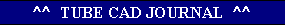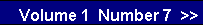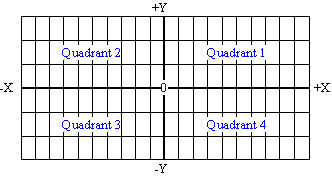Plate Curves -Part 1-
 We call his grid a system of rectangular coordinates. It defines four rectangular sections or quadrants. Fortunately, we only need only the top right quadrant to map out the usual grid voltage lines that make up the tube manual IV (current/voltage) curves. This is so because tubes are unidirectional current conducting devices: they only conduct from cathode to plate. But before we tackle the vacuum tube's plate curves, we need to cover something much simpler: resistance and straight lines.One Resistance   The movement from the graph's left to right defines increasing voltage; from bottom to top, increasing current. This arrangement allows us to graphically to display Ohm's Law: Resistance = Voltage / Current. For example, 1 ohm equals 1 volt divided by 1 amp and 100 ohms equals 100 volt divided by 1 amp. Graphing these resistances is easy. We know we must start at the bottom left corner where voltage and current equal zero, as no voltage across a resistance means no current. The next point for the 1 ohm resistance would be one unit  to the right and one unit up and for the 100 ohm resistance it would be one hundred units to the right and one units up. Remember, the lines continue infinitely, as the relationship between voltage and current defined by the line is constant.  So if we follow the 1 ohm line out to 100, 000 volts, we know that the current will be 100, 000 amps. Of course, no 1 ohm resistor on earth could withstand the test; but the issue here was resistance and not necessarily actual resistors.   From the plotting of these two resistances, a key feature of graphing resistances is revealed: the lower the resistance, the steeper the line defined; and the higher  the resistance, the less steep the line defined. (This will prove valuable when working with tubes, as the principle allows quick inspection of plate resistances, rp's. Given that both graphs have the same X-Y scales, the steeper set of plate curves belongs to
 The FBI agent has finger prints; the fortune teller, a crystal ball; and the tube amplifier designer, plate curves. Each promises to reveal secrets. Who did it? What will future bring? Will it work? Like the finger print, the tube's plate curves individualize the tube, which helps us decide which tube should be used. Like the crystal ball, a set of plate curves helps us predict how an amplifier will function. So important are the plate curves to tube audio design that really everyone working with vacuum tubes should be able to quickly find the required grid voltage to set a given bias point and be able to determine maximum voltage swings. Yet, many do not understand what the curves mean or how to use them. When showed a the plate curves of a particularly non-linear tube, a friend of mine said, "Wow, that's linear." Surprised by his remark I asked, "How so?" His reply was, "The lines are so straight."First the History No One Reads   Renee Descartes deserves the credit for the XY graph. Almost delirious, exhausted, he lay sick in bed, staring at the bars that covered his window. Then it hit him: if center point was defined as zero and a string of positive numbers were ordered to the right and then again up from the center, while a string of negative numbers were ordered to the left and then again below the center, any two numbers would define a point on this grid. This was the beginning of analytic geometry.pg. 6